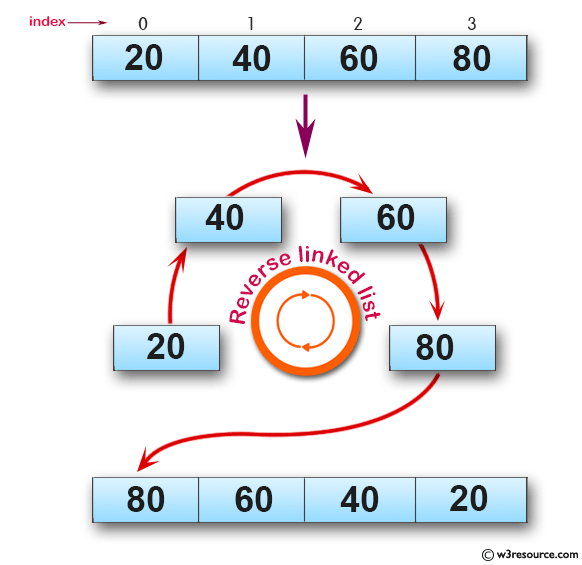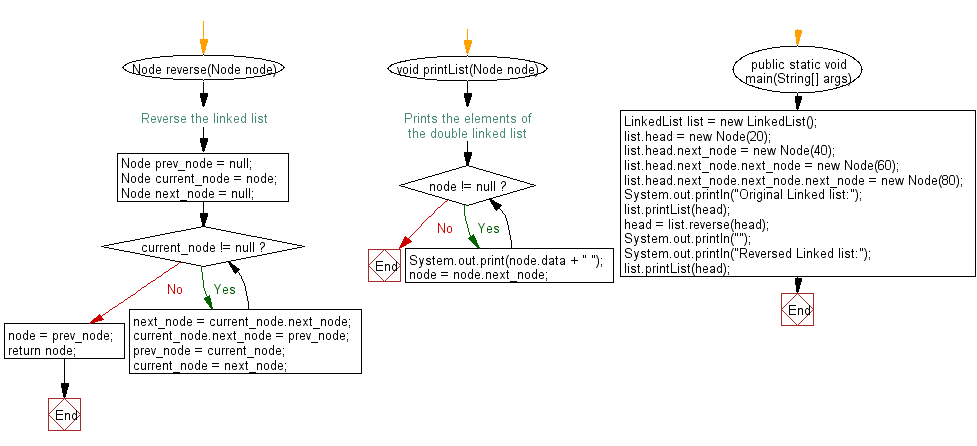﻿ Java exercises: Reverse a given linked list - w3resource# Java Exercises: Reverse a given linked list

## Java Basic: Exercise-121 with Solution

Write a Java program to reverse a given linked list.

Pictorial Presentation:Sample Solution:

Java Code:

``````class LinkedList {

static class Node {

int data;
Node next_node;

Node(int d) {
data = d;
next_node = null;
}
}

/* Reverse the linked list */
Node reverse(Node node) {
Node prev_node = null;
Node current_node = node;
Node next_node = null;
while (current_node != null) {
next_node = current_node.next_node;
current_node.next_node = prev_node;
prev_node = current_node;
current_node = next_node;
}
node = prev_node;
return node;
}

// Prints the elements of the double linked list
void printList(Node node) {
while (node != null) {
System.out.print(node.data + " ");
node = node.next_node;
}
}

public static void main(String[] args) {

System.out.println("");
}
}
```
```

Sample Output:

```Original Linked list:
20 40 60 80
80 60 40 20
```

Flowchart:Java Code Editor:

What is the difficulty level of this exercise?

Test your Programming skills with w3resource's quiz.

﻿

## Java: Tips of the Day

countOccurrences

Counts the occurrences of a value in an array.

Use Arrays.stream().filter().count() to count total number of values that equals the specified value.

```public static long countOccurrences(int[] numbers, int value) {
return Arrays.stream(numbers)
.filter(number -> number == value)
.count();
}
```

Ref: https://bit.ly/3kCAgLb